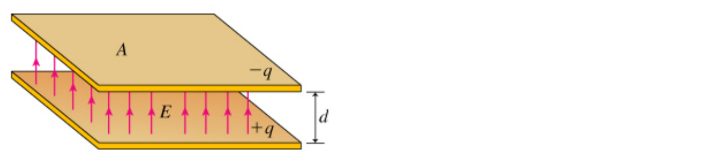# Problem: Parts (A) What property of objects is best measured by their capacitance?a. the ability to conduct electric currentb. the ability to distort an external electrostatic fieldc. the ability to store charge Part (B) Assume that charge -q is placed on the top plate, and +q is placed on the bottom plate. What is the magnitude of the electric field E between the plates? Express E in terms of q and other quantities given in the introduction, in addition to ε0 and any other constants needed. Part (C) What is the voltage V between the plates of the capacitor? Express V in terms of the quantities given in the introduction and any required physical constants. Part (D) Now find the capacitance C of the parallel-plate capacitor. Express C in terms of quantities given in the introduction and constants like ε0. Part (E) Consider an air-filled charged capacitor. How can its capacitance be increased?a. Increase the charge on the capacitor.b. Decrease the charge on the capacitor.c. Increase the spacing between the plates of the capacitor.d. Decrease the spacing between the plates of the capacitor.e. Increase the length of the wires leading to the capacitor plates. Part (F) Consider a charged parallel-plate capacitor. How can its capacitance be halved? Check all that apply.a. Double the charge.b. Double the plate area.c. Double the plate separation.d. Halve the charge.e. Halve the plate area.f. Halve the plate separationPart (G) Consider a charged parallel-plate capacitor. Which combination of changes would quadruple its capacitance?a. Double the charge and double the plate area.b. Double the charge and double the plate separation.d. Halve the charge and double the plate separation.e. Halve the charge and double the plate area.f. Halve the plate separation and double the plate area.g. Double the plate separation and halve the plate area.

###### FREE Expert Solution

Parts (A)

A capacitor is known to store charge.

97% (157 ratings)###### Problem Details

Parts (A) What property of objects is best measured by their capacitance?

a. the ability to conduct electric current

b. the ability to distort an external electrostatic field

c. the ability to store charge

Part (B) Assume that charge -q is placed on the top plate, and +q is placed on the bottom plate. What is the magnitude of the electric field E between the plates? Express E in terms of q and other quantities given in the introduction, in addition to ε0 and any other constants needed.

Part (C) What is the voltage V between the plates of the capacitor? Express V in terms of the quantities given in the introduction and any required physical constants.

Part (D) Now find the capacitance C of the parallel-plate capacitor. Express C in terms of quantities given in the introduction and constants like ε0.

Part (E) Consider an air-filled charged capacitor. How can its capacitance be increased?

a. Increase the charge on the capacitor.

b. Decrease the charge on the capacitor.

c. Increase the spacing between the plates of the capacitor.

d. Decrease the spacing between the plates of the capacitor.

e. Increase the length of the wires leading to the capacitor plates.

Part (F) Consider a charged parallel-plate capacitor. How can its capacitance be halved? Check all that apply.

a. Double the charge.
b. Double the plate area.
c. Double the plate separation.
d. Halve the charge.
e. Halve the plate area.
f. Halve the plate separation

Part (G) Consider a charged parallel-plate capacitor. Which combination of changes would quadruple its capacitance?

a. Double the charge and double the plate area.

b. Double the charge and double the plate separation.

d. Halve the charge and double the plate separation.

e. Halve the charge and double the plate area.

f. Halve the plate separation and double the plate area.

g. Double the plate separation and halve the plate area.